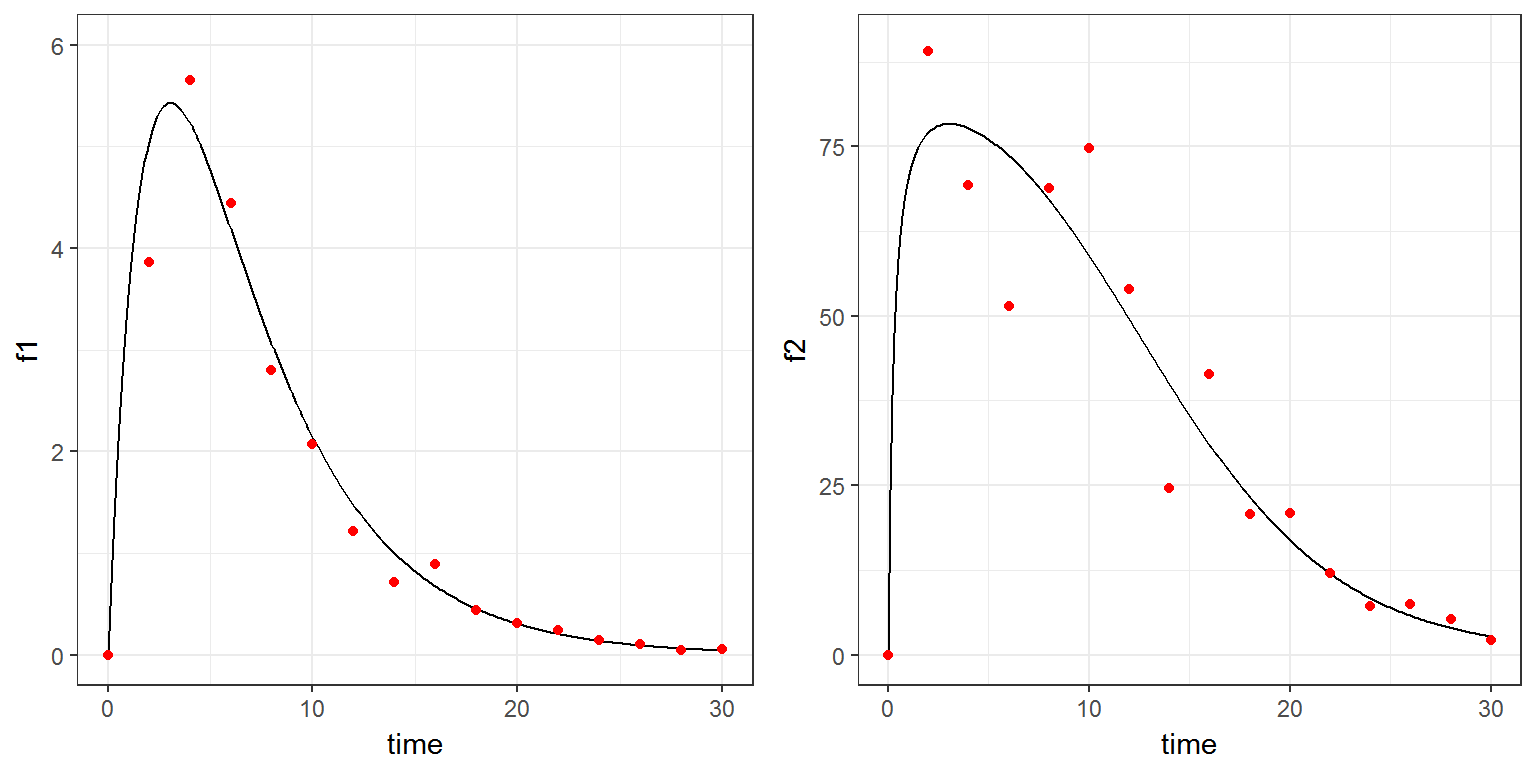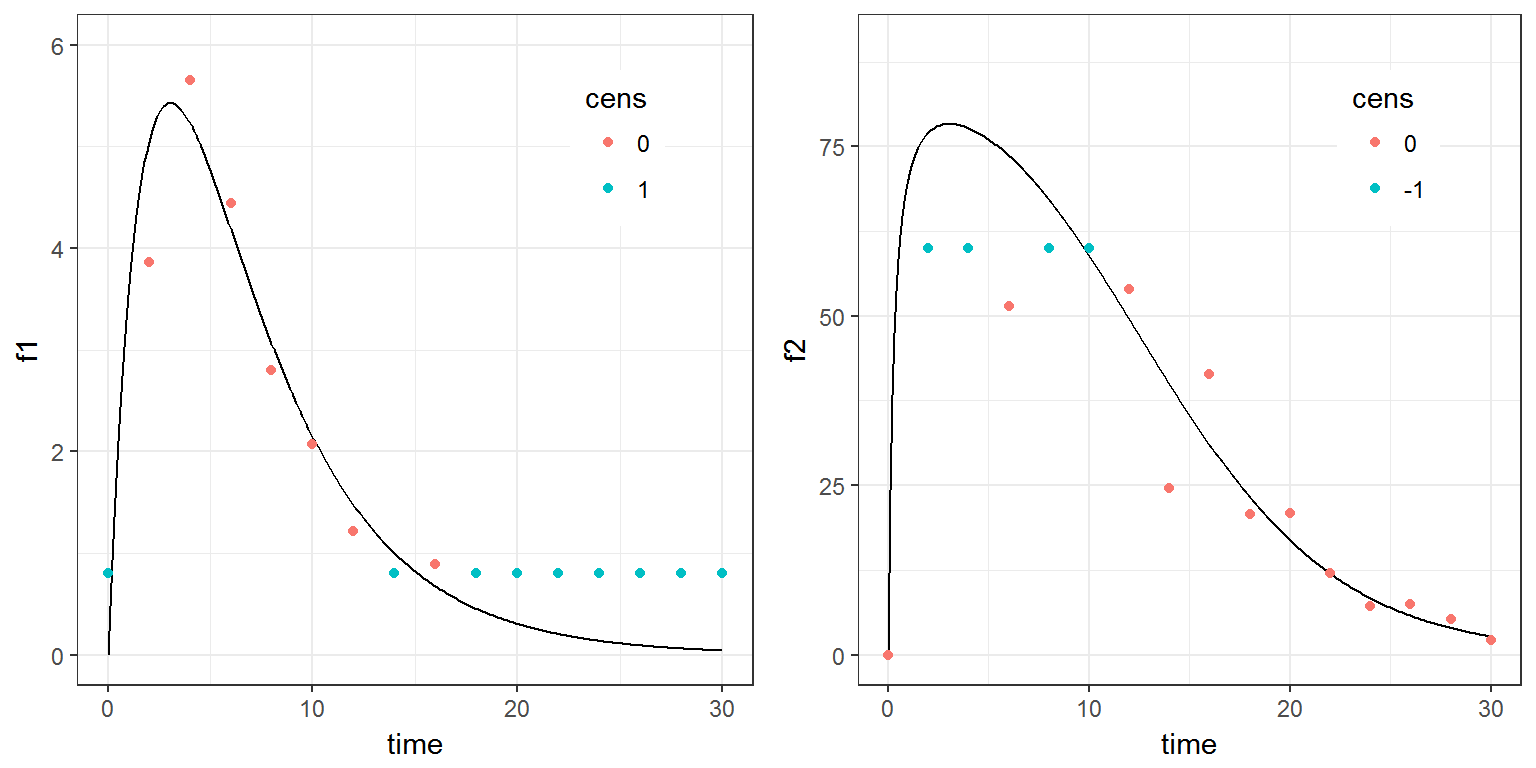R script: censored.R

$$\newcommand{\esp}{\mathbb{E}\left(#1\right)} \newcommand{\var}{\mbox{Var}\left(#1\right)} \newcommand{\deriv}{\dot{#1}(t)} \newcommand{\prob}{ \mathbb{P}\!(#1)} \newcommand{\eqdef}{\mathop{=}\limits^{\mathrm{def}}} \newcommand{\by}{\boldsymbol{y}} \newcommand{\bc}{\boldsymbol{c}} \newcommand{\bpsi}{\boldsymbol{\psi}} \def\pmacro{\texttt{p}} \def\like{{\cal L}} \def\llike{{\cal LL}} \def\logit{{\rm logit}} \def\probit{{\rm probit}} \def\one{{\rm 1\!I}} \def\iid{\mathop{\sim}_{\rm i.i.d.}} \def\simh0{\mathop{\sim}_{H_0}} \def\df{\texttt{df}} \def\res{e} \def\xomega{x} \newcommand{\argmin}{{\rm arg}\min_{#1}} \newcommand{\argmax}{{\rm arg}\max_{#1}} \newcommand{\Rset}{\mbox{\mathbb{R}}} \def\param{\theta} \def\setparam{\Theta} \def\xnew{x_{\rm new}} \def\fnew{f_{\rm new}} \def\ynew{y_{\rm new}} \def\nnew{n_{\rm new}} \def\enew{e_{\rm new}} \def\Xnew{X_{\rm new}} \def\hfnew{\widehat{\fnew}} \def\degree{m} \def\nbeta{d} \newcommand{\limite}{\mathop{\longrightarrow}\limits_{#1}} \def\ka{k{a}} \def\ska{k{\scriptscriptstyle a}} \def\kel{k{e}} \def\skel{k{\scriptscriptstyle e}} \def\cl{C{\small l}} \def\Tlag{T\hspace{-0.1em}{lag}} \def\sTlag{T\hspace{-0.07em}{\scriptscriptstyle lag}} \def\Tk{T\hspace{-0.1em}{k0}} \def\sTk{T\hspace{-0.07em}{\scriptscriptstyle k0}} \def\thalf{t{1/2}} \newcommand{\Dphi}{\partial_\pphi #1} \def\asigma{a} \def\pphi{\psi} \newcommand{\stheta}{{\theta^\star}} \newcommand{\htheta}{{\widehat{\theta}}}$$

# 1 Introduction

Censoring occurs when the value of a measurement or observation is only partially known. In the longitudinal context, censoring refers to the values of the measurements, not the times at which they were taken.

For example, the lower limit of quantification (LLOQ) is the lowest quantity of a substance that can be reliably measured. Therefore, any time the quantity is below the LLOQ, the observation’’ is not a measurement but the information that the measured quantity is less than the LLOQ.

A measuring device can also have an upper limit of quantification (ULOQ) such that any value above this limit cannot be measured and reported.

As hinted above, censored values are not typically reported as a number, but their existence is known, as well as the type of censoring. Thus, the observation $$y_{ij}^{(r)}$$ (i.e., what is reported) is the measurement $$y_{ij}$$ if not censored, and the type of censoring otherwise. We usually distinguish between three types of censoring: left, right and interval.

# 2 Example

We use here a basic model for continuous PKPD data:

myModel <- inlineModel("
[LONGITUDINAL]
input = {ka, V, k, Emax, EC50, b1, b2}

EQUATION:
D = 100
f1 = D*ka/(V*(ka-k))*(exp(-k*t) - exp(-ka*t))
f2 = Emax*f1/(f1 + EC50)
g1 = b1*f1
g2 = b2*f2

DEFINITION:
y1 = {distribution=normal, prediction=f1, sd=g1}
y2 = {distribution=normal, prediction=f2, sd=g2}
")

We will start evaluating the predictions $$f_1$$ and $$f_2$$ and simulating 2 sequences of observations $$y_1$$ and $$y_2$$:

f <- list(name= c('f1','f2'), time=seq(0, 30, by=0.1))
y <- list(name=c('y1','y2'), time=seq(0, 30, by=2))
p <- c(ka=0.5, V=10, k=0.2, Emax=100, EC50=1.5, b1=0.2, b2=0.15)
s <- 123456

res1a <- simulx(model   = myModel,
parameter = p,
output    = list(f, y),
settings  = list(seed=s) )

library(gridExtra)
pla1 <- ggplot() + geom_line(data=res1a$f1, aes(x=time, y=f1), size=0.5) + geom_point(data=res1a$y1, aes(x=time, y=y1), colour="red") + ylim(c(0, 6))
pla2 <- ggplot() + geom_line(data=res1a$f2, aes(x=time, y=f2), size=0.5) + geom_point(data=res1a$y2, aes(x=time, y=y2), colour="red") + ylim(c(0, 90))
grid.arrange(pla1, pla2, ncol=2)We now introduce a lower limit of quantification LLOQ=1.8 for the PK data and an upper limit for the PD data ULOQ=60:

y1 <- list(name='y1', time=seq(0, 30, by=2), lloq=0.8)
y2 <- list(name='y2', time=seq(0, 30, by=2), uloq=60)

res1b <- simulx(model   = myModel,
parameter = p,
output    = list(y1, y2),
settings  = list(seed=s) )

Simulx creates a column CENS that takes the value 0 when the data is not censored, 1 when the data is left censored (BLQ data) and -1 if the data is right censored (ALQ data).

head(res1b$y1) ## time y1 cens ## 1 0 0.800000 1 ## 2 2 3.865404 0 ## 3 4 5.649620 0 ## 4 6 4.443873 0 ## 5 8 2.795366 0 ## 6 10 2.074896 0 head(res1b$y2)
##   time       y2 cens
## 1    0  0.00000    0
## 2    2 60.00000   -1
## 3    4 60.00000   -1
## 4    6 51.50381    0
## 5    8 60.00000   -1
## 6   10 60.00000   -1
plb1 <- ggplot() + geom_line(data=res1a$f1, aes(x=time, y=f1), size=0.5) + geom_point(data=res1b$y1, aes(x=time, y=y1, colour=cens)) +
theme(legend.position=c(.8, .8)) + ylim(c(0, 6))
plb2 <- ggplot() + geom_line(data=res1a$f2, aes(x=time, y=f2), size=0.5) + geom_point(data=res1b$y2, aes(x=time, y=y2, colour=cens))+
theme(legend.position=c(.8, .8)) + ylim(c(0, 90))
grid.arrange(plb1, plb2, ncol=2)In addition, we can take into account the fact that a concentration only takes positive values. In other word, a concentration below the limit of quantification (BLQ data) is known to be between 0 and LLOQ. The lower limit of the censoring interval is then defined as LIMIT=0.

y1$limit=0 res1b <- simulx(model = myModel, parameter = p, output = list(y1, y2), settings = list(seed=s) ) An additional column LIMIT is then created: head(res1b$y1)
##   time       y1 cens limit
## 1    0 0.800000    1     0
## 2    2 3.865404    0     0
## 3    4 5.649620    0     0
## 4    6 4.443873    0     0
## 5    8 2.795366    0     0
## 6   10 2.074896    0     0

The simulated data can be saved in a single file using the Monolix format (see the Monolix documentation for more information).

writeDatamlx(res1b, result.file = "res1b.csv")
head(read.table("res1b.csv", header=T, sep=","), n=20)
##    time        y cens limit ytype
## 1     0  0.80000    1     0     1
## 2     0  0.00000    0     .     2
## 3     2  3.86540    0     .     1
## 4     2 60.00000   -1     .     2
## 5     4  5.64962    0     .     1
## 6     4 60.00000   -1     .     2
## 7     6  4.44387    0     .     1
## 8     6 51.50381    0     .     2
## 9     8  2.79537    0     .     1
## 10    8 60.00000   -1     .     2
## 11   10  2.07490    0     .     1
## 12   10 60.00000   -1     .     2
## 13   12  1.21758    0     .     1
## 14   12 53.88158    0     .     2
## 15   14  0.80000    1     0     1
## 16   14 24.52708    0     .     2
## 17   16  0.88724    0     .     1
## 18   16 41.39897    0     .     2
## 19   18  0.80000    1     0     1
## 20   18 20.79786    0     .     2

The file can also be created directly from Simulx:

res1c <- simulx(model     = myModel,
parameter = p,
result.file   ="res1c.csv",
output    = list(y1, y2),
settings  = list(seed=s))

head(read.table("res1c.csv", header=T, sep=","))
##   time       y cens limit ytype
## 1    0  0.8000    1     0     1
## 2    0  0.0000    0     .     2
## 3    2  3.8654    0     .     1
## 4    2 60.0000   -1     .     2
## 5    4  5.6496    0     .     1
## 6    4 60.0000   -1     .     2